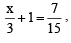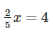Courses

# Linear Equations In One Variable - MCQ

## 10 Questions MCQ Test Class 8 Mathematics by VP Classes | Linear Equations In One Variable - MCQ

Description
This mock test of Linear Equations In One Variable - MCQ for Class 8 helps you for every Class 8 entrance exam. This contains 10 Multiple Choice Questions for Class 8 Linear Equations In One Variable - MCQ (mcq) to study with solutions a complete question bank. The solved questions answers in this Linear Equations In One Variable - MCQ quiz give you a good mix of easy questions and tough questions. Class 8 students definitely take this Linear Equations In One Variable - MCQ exercise for a better result in the exam. You can find other Linear Equations In One Variable - MCQ extra questions, long questions & short questions for Class 8 on EduRev as well by searching above.
QUESTION: 1

Solution:
QUESTION: 2

Solution:
QUESTION: 3

### If, then which of the following correct?

Solution:
QUESTION: 4

If 7x + 15 = 50, then which of the following is the root of the equation?

Solution:
QUESTION: 5

Ifthen which of the following is the value of x?

Solution:
QUESTION: 6

In the sum of two consecutive numbers is 71 and one of them being x, then which of the following is the other number?

Solution:
QUESTION: 7

Two years ago my age was ‘x’ years 5 years ago my age was?

Solution:
X-3
present age=x+2
before 5 years=x+2-5=x-3
QUESTION: 8

10 years ago I was ‘x’ years old. After 10 years, my age will be:

Solution:
QUESTION: 9

In 15/4 – 7x = 9, x=

Solution:
QUESTION: 10

If ‘x’ is an even number, then which of the following is the next odd number is?

Solution: